# 10.3 Polar coordinates  (Page 5/8)

 Page 5 / 8

How are the polar axes different from the x - and y -axes of the Cartesian plane?

Explain how polar coordinates are graphed.

Determine $\text{\hspace{0.17em}}\theta \text{\hspace{0.17em}}$ for the point, then move $\text{\hspace{0.17em}}r\text{\hspace{0.17em}}$ units from the pole to plot the point. If $\text{\hspace{0.17em}}r\text{\hspace{0.17em}}$ is negative, move $\text{\hspace{0.17em}}r\text{\hspace{0.17em}}$ units from the pole in the opposite direction but along the same angle. The point is a distance of $\text{\hspace{0.17em}}r\text{\hspace{0.17em}}$ away from the origin at an angle of $\text{\hspace{0.17em}}\theta \text{\hspace{0.17em}}$ from the polar axis.

How are the points $\text{\hspace{0.17em}}\left(3,\frac{\pi }{2}\right)\text{\hspace{0.17em}}$ and $\text{\hspace{0.17em}}\left(-3,\frac{\pi }{2}\right)\text{\hspace{0.17em}}$ related?

Explain why the points $\text{\hspace{0.17em}}\left(-3,\frac{\pi }{2}\right)\text{\hspace{0.17em}}$ and $\text{\hspace{0.17em}}\left(3,-\frac{\pi }{2}\right)\text{\hspace{0.17em}}$ are the same.

The point $\text{\hspace{0.17em}}\left(-3,\frac{\pi }{2}\right)\text{\hspace{0.17em}}$ has a positive angle but a negative radius and is plotted by moving to an angle of $\text{\hspace{0.17em}}\frac{\pi }{2}\text{\hspace{0.17em}}$ and then moving 3 units in the negative direction. This places the point 3 units down the negative y -axis. The point $\text{\hspace{0.17em}}\left(3,-\frac{\pi }{2}\right)\text{\hspace{0.17em}}$ has a negative angle and a positive radius and is plotted by first moving to an angle of $\text{\hspace{0.17em}}-\frac{\pi }{2}\text{\hspace{0.17em}}$ and then moving 3 units down, which is the positive direction for a negative angle. The point is also 3 units down the negative y -axis.

## Algebraic

For the following exercises, convert the given polar coordinates to Cartesian coordinates with $\text{\hspace{0.17em}}r>0\text{\hspace{0.17em}}$ and $\text{\hspace{0.17em}}0\le \theta \le 2\pi .\text{\hspace{0.17em}}$ Remember to consider the quadrant in which the given point is located when determining $\text{\hspace{0.17em}}\theta \text{\hspace{0.17em}}$ for the point.

$\left(7,\frac{7\pi }{6}\right)$

$\left(5,\pi \right)$

$\left(-5,0\right)$

$\left(6,-\frac{\pi }{4}\right)$

$\left(-3,\frac{\pi }{6}\right)$

$\left(-\frac{3\sqrt{3}}{2},-\frac{3}{2}\right)$

$\left(4,\frac{7\pi }{4}\right)$

For the following exercises, convert the given Cartesian coordinates to polar coordinates with $\text{\hspace{0.17em}}r>0,\text{\hspace{0.17em}}\text{\hspace{0.17em}}0\le \theta <2\pi .\text{\hspace{0.17em}}$ Remember to consider the quadrant in which the given point is located.

$\left(4,2\right)$

$\left(-4,6\right)$

$\left(3,-5\right)$

$\left(\sqrt{34},5.253\right)$

$\left(-10,-13\right)$

$\left(8,8\right)$

$\left(8\sqrt{2},\frac{\pi }{4}\right)$

For the following exercises, convert the given Cartesian equation to a polar equation.

$x=3$

$y=4$

$r=4\mathrm{csc}\theta$

$y=4{x}^{2}$

$y=2{x}^{4}$

$r=\sqrt{\frac{sin\theta }{2co{s}^{4}\theta }}$

${x}^{2}+{y}^{2}=4y$

${x}^{2}+{y}^{2}=3x$

$r=3\mathrm{cos}\theta$

${x}^{2}-{y}^{2}=x$

${x}^{2}-{y}^{2}=3y$

$r=\frac{3\mathrm{sin}\theta }{\mathrm{cos}\left(2\theta \right)}$

${x}^{2}+{y}^{2}=9$

${x}^{2}=9y$

$r=\frac{9\mathrm{sin}\theta }{{\mathrm{cos}}^{2}\theta }$

${y}^{2}=9x$

$9xy=1$

$r=\sqrt{\frac{1}{9\mathrm{cos}\theta \mathrm{sin}\theta }}$

For the following exercises, convert the given polar equation to a Cartesian equation. Write in the standard form of a conic if possible, and identify the conic section represented.

$r=3\mathrm{sin}\text{\hspace{0.17em}}\theta$

$r=4\mathrm{cos}\text{\hspace{0.17em}}\theta$

${x}^{2}+{y}^{2}=4x\text{\hspace{0.17em}}$ or $\text{\hspace{0.17em}}\frac{{\left(x-2\right)}^{2}}{4}+\frac{{y}^{2}}{4}=1;$ circle

$r=\frac{4}{\mathrm{sin}\text{\hspace{0.17em}}\theta +7\mathrm{cos}\text{\hspace{0.17em}}\theta }$

$r=\frac{6}{\mathrm{cos}\text{\hspace{0.17em}}\theta +3\mathrm{sin}\text{\hspace{0.17em}}\theta }$

$3y+x=6;\text{\hspace{0.17em}}$ line

$r=2\mathrm{sec}\text{\hspace{0.17em}}\theta$

$r=3\mathrm{csc}\text{\hspace{0.17em}}\theta$

$y=3;\text{\hspace{0.17em}}$ line

$r=\sqrt{r\mathrm{cos}\text{\hspace{0.17em}}\theta +2}$

${r}^{2}=4\mathrm{sec}\text{\hspace{0.17em}}\theta \text{\hspace{0.17em}}\mathrm{csc}\text{\hspace{0.17em}}\theta$

$xy=4;\text{\hspace{0.17em}}$ hyperbola

$r=4$

${r}^{2}=4$

${x}^{2}+{y}^{2}=4;\text{\hspace{0.17em}}$ circle

$r=\frac{1}{4\mathrm{cos}\text{\hspace{0.17em}}\theta -3\mathrm{sin}\text{\hspace{0.17em}}\theta }$

$r=\frac{3}{\mathrm{cos}\text{\hspace{0.17em}}\theta -5\mathrm{sin}\text{\hspace{0.17em}}\theta }$

$x-5y=3;\text{\hspace{0.17em}}$ line

## Graphical

For the following exercises, find the polar coordinates of the point.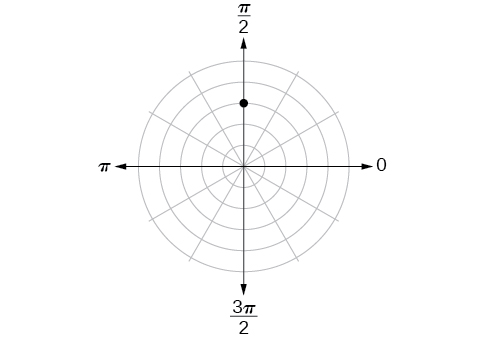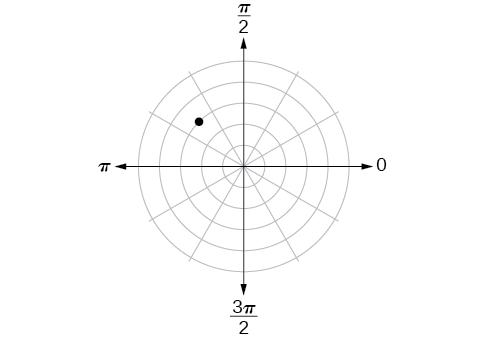$\left(3,\frac{3\pi }{4}\right)$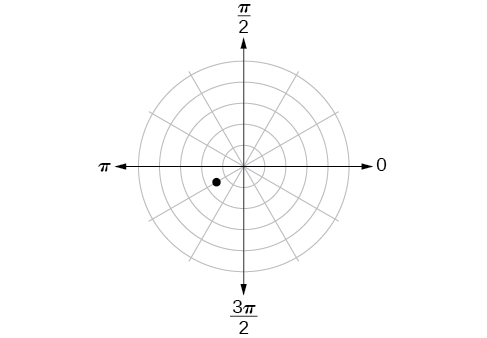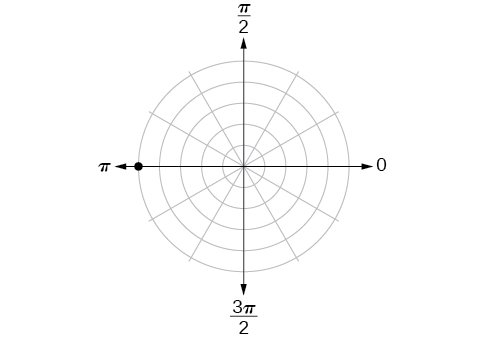$\left(5,\pi \right)$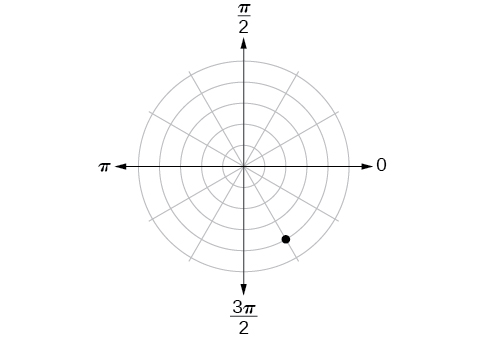For the following exercises, plot the points.

$\left(-2,\frac{\pi }{3}\right)$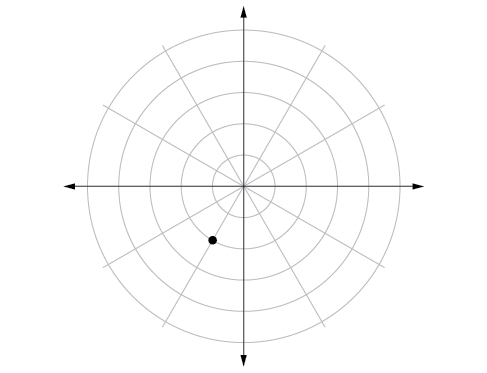$\left(-1,-\frac{\pi }{2}\right)$

$\left(3.5,\frac{7\pi }{4}\right)$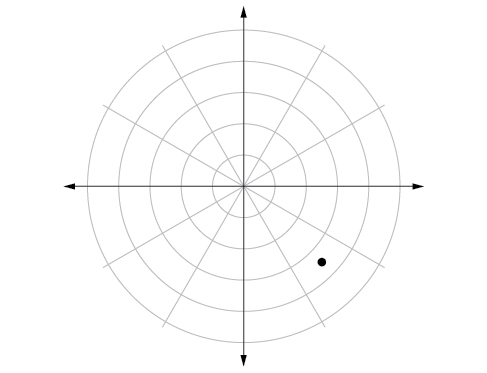$\left(-4,\frac{\pi }{3}\right)$

$\left(5,\frac{\pi }{2}\right)$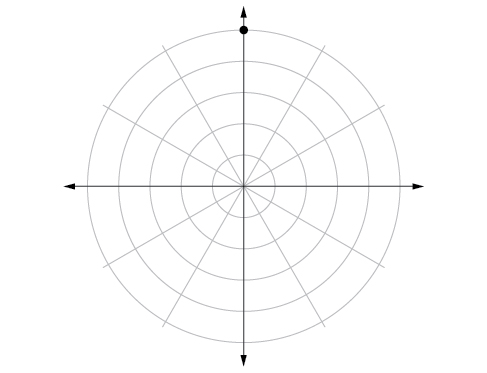$\left(4,\frac{-5\pi }{4}\right)$

$\left(3,\frac{5\pi }{6}\right)$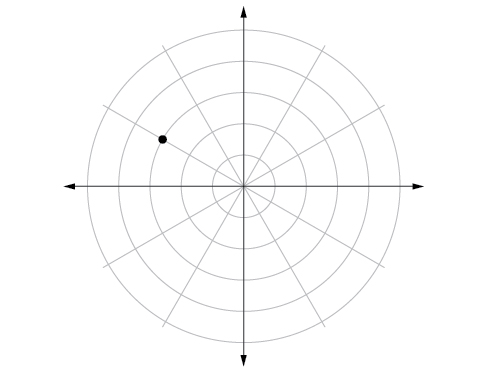$\left(-1.5,\frac{7\pi }{6}\right)$

$\left(-2,\frac{\pi }{4}\right)$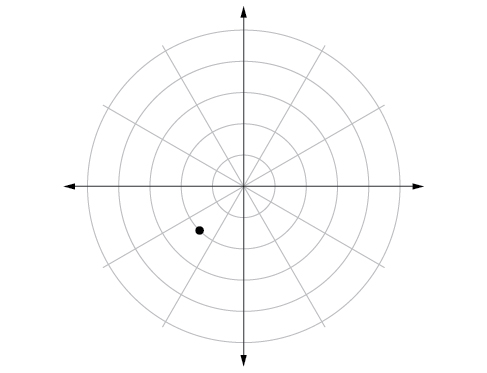$\left(1,\frac{3\pi }{2}\right)$

For the following exercises, convert the equation from rectangular to polar form and graph on the polar axis.

$5x-y=6$

$r=\frac{6}{5\mathrm{cos}\theta -\mathrm{sin}\theta }$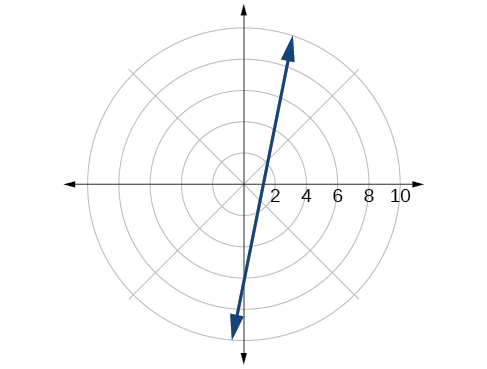$2x+7y=-3$

${x}^{2}+{\left(y-1\right)}^{2}=1$

$r=2\mathrm{sin}\theta$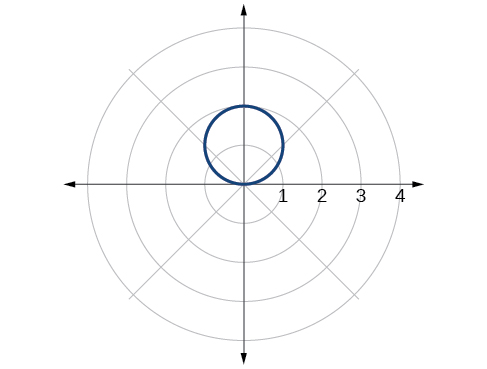${\left(x+2\right)}^{2}+{\left(y+3\right)}^{2}=13$

$x=2$

$r=\frac{2}{\mathrm{cos}\theta }$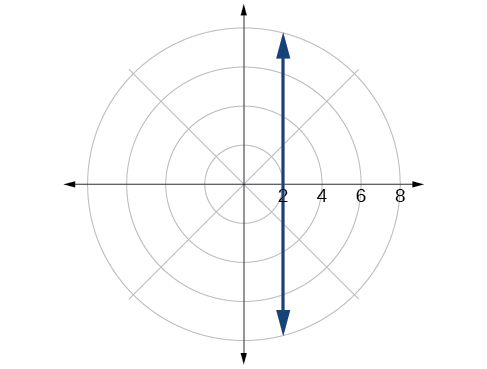${x}^{2}+{y}^{2}=5y$

${x}^{2}+{y}^{2}=3x$

$r=3\mathrm{cos}\theta$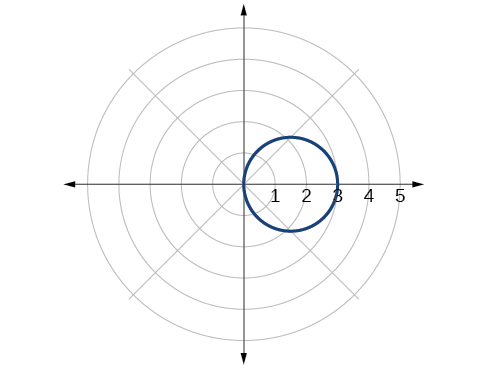For the following exercises, convert the equation from polar to rectangular form and graph on the rectangular plane.

$r=6$

$r=-4$

${x}^{2}+{y}^{2}=16$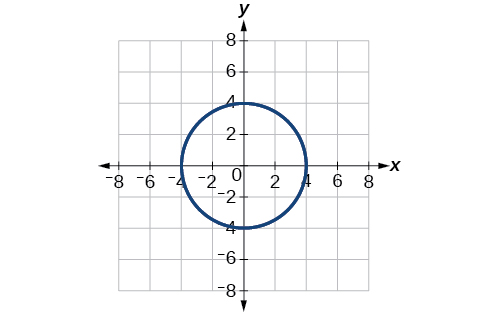$\theta =-\frac{2\pi }{3}$

$\theta =\frac{\pi }{4}$

$y=x$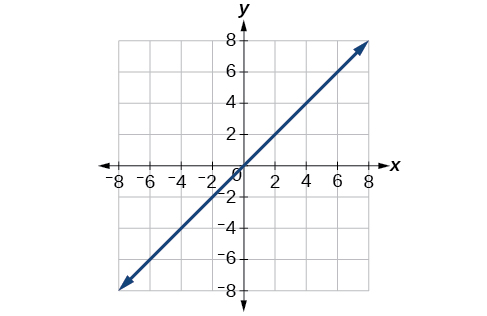$r=\mathrm{sec}\text{\hspace{0.17em}}\theta$

$r=-10\mathrm{sin}\text{\hspace{0.17em}}\theta$

${x}^{2}+{\left(y+5\right)}^{2}=25$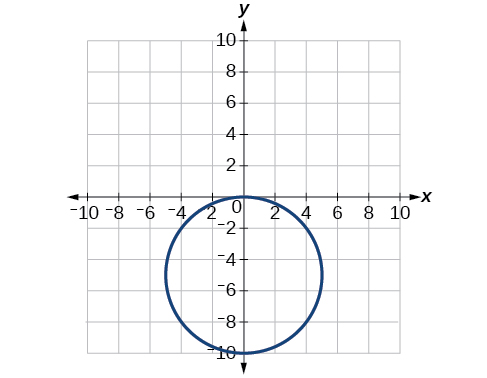$r=3\mathrm{cos}\text{\hspace{0.17em}}\theta$

## Technology

Use a graphing calculator to find the rectangular coordinates of $\text{\hspace{0.17em}}\left(2,-\frac{\pi }{5}\right).\text{\hspace{0.17em}}$ Round to the nearest thousandth.

$\left(1.618,-1.176\right)$

Use a graphing calculator to find the rectangular coordinates of $\text{\hspace{0.17em}}\left(-3,\frac{3\pi }{7}\right).\text{\hspace{0.17em}}$ Round to the nearest thousandth.

Use a graphing calculator to find the polar coordinates of $\text{\hspace{0.17em}}\left(-7,8\right)\text{\hspace{0.17em}}$ in degrees. Round to the nearest thousandth.

$\left(10.630,131.186°\right)$

Use a graphing calculator to find the polar coordinates of $\text{\hspace{0.17em}}\left(3,-4\right)\text{\hspace{0.17em}}$ in degrees. Round to the nearest hundredth.

Use a graphing calculator to find the polar coordinates of $\text{\hspace{0.17em}}\left(-2,0\right)\text{\hspace{0.17em}}$ in radians. Round to the nearest hundredth.

$\text{\hspace{0.17em}}\left(2,3.14\right)or\left(2,\pi \right)\text{\hspace{0.17em}}$

## Extensions

Describe the graph of $\text{\hspace{0.17em}}r=a\mathrm{sec}\text{\hspace{0.17em}}\theta ;a>0.$

Describe the graph of $\text{\hspace{0.17em}}r=a\mathrm{sec}\text{\hspace{0.17em}}\theta ;a<0.$

A vertical line with $\text{\hspace{0.17em}}a\text{\hspace{0.17em}}$ units left of the y -axis.

Describe the graph of $\text{\hspace{0.17em}}r=a\mathrm{csc}\text{\hspace{0.17em}}\theta ;a>0.$

Describe the graph of $\text{\hspace{0.17em}}r=a\mathrm{csc}\text{\hspace{0.17em}}\theta ;a<0.$

A horizontal line with $\text{\hspace{0.17em}}a\text{\hspace{0.17em}}$ units below the x -axis.

What polar equations will give an oblique line?

For the following exercise, graph the polar inequality.

$r<4$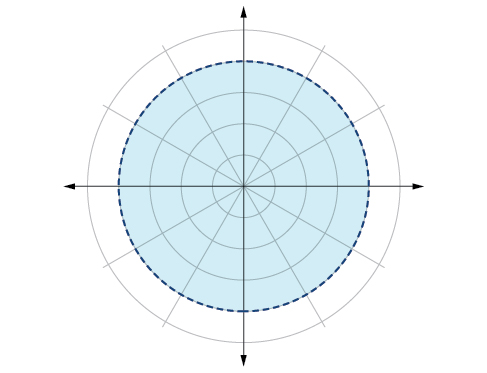$0\le \theta \le \frac{\pi }{4}$

$\theta =\frac{\pi }{4},\text{\hspace{0.17em}}r\text{\hspace{0.17em}}\ge \text{\hspace{0.17em}}2$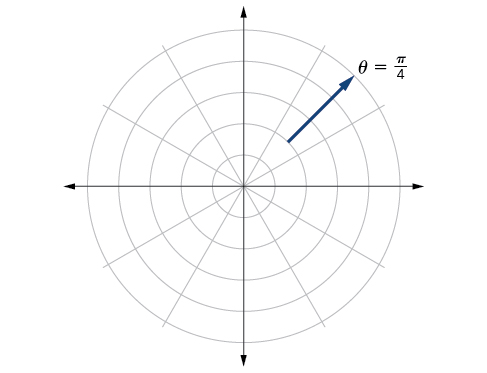$\theta =\frac{\pi }{4},\text{\hspace{0.17em}}r\text{\hspace{0.17em}}\ge -3$

$0\le \theta \le \frac{\pi }{3},\text{\hspace{0.17em}}r\text{\hspace{0.17em}}<\text{\hspace{0.17em}}2$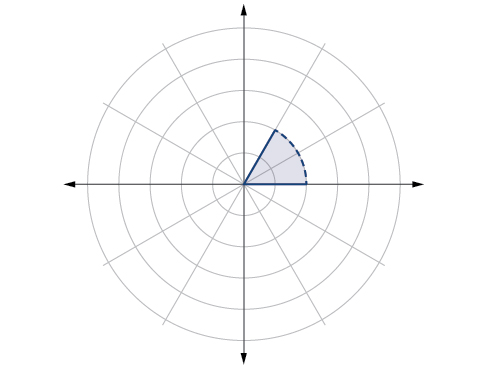$\frac{-\pi }{6}<\theta \le \frac{\pi }{3},-3

answer and questions in exercise 11.2 sums
how do u calculate inequality of irrational number?
Alaba
give me an example
Chris
and I will walk you through it
Chris
cos (-z)= cos z .
what is a algebra
(x+x)3=?
what is the identity of 1-cos²5x equal to?
__john __05
Kishu
Hi
Abdel
hi
Ye
hi
Nokwanda
C'est comment
Abdel
Hi
Amanda
hello
SORIE
Hiiii
Chinni
hello
Ranjay
hi
ANSHU
hiiii
Chinni
h r u friends
Chinni
yes
Hassan
so is their any Genius in mathematics here let chat guys and get to know each other's
SORIE
I speak French
Abdel
okay no problem since we gather here and get to know each other
SORIE
hi im stupid at math and just wanna join here
Yaona
lol nahhh none of us here are stupid it's just that we have Fast, Medium, and slow learner bro but we all going to work things out together
SORIE
it's 12
what is the function of sine with respect of cosine , graphically
tangent bruh
Steve
cosx.cos2x.cos4x.cos8x
sinx sin2x is linearly dependent
what is a reciprocal
The reciprocal of a number is 1 divided by a number. eg the reciprocal of 10 is 1/10 which is 0.1
Shemmy
Reciprocal is a pair of numbers that, when multiplied together, equal to 1. Example; the reciprocal of 3 is ⅓, because 3 multiplied by ⅓ is equal to 1
Jeza
each term in a sequence below is five times the previous term what is the eighth term in the sequence
I don't understand how radicals works pls
How look for the general solution of a trig function
stock therom F=(x2+y2) i-2xy J jaha x=a y=o y=b
sinx sin2x is linearly dependent
cr
root under 3-root under 2 by 5 y square
The sum of the first n terms of a certain series is 2^n-1, Show that , this series is Geometric and Find the formula of the n^th
cosA\1+sinA=secA-tanA
Wrong question
why two x + seven is equal to nineteen.
The numbers cannot be combined with the x
Othman
2x + 7 =19
humberto
2x +7=19. 2x=19 - 7 2x=12 x=6
Yvonne
because x is 6
SAIDIByByBy Jonathan LongBy Kimberly NicholsBy OpenStaxBy Hope PercleBy Steve GibbsBy OpenStaxBy Richley CrapoBy DanielrosenbergerBy Dewey ComptonBy